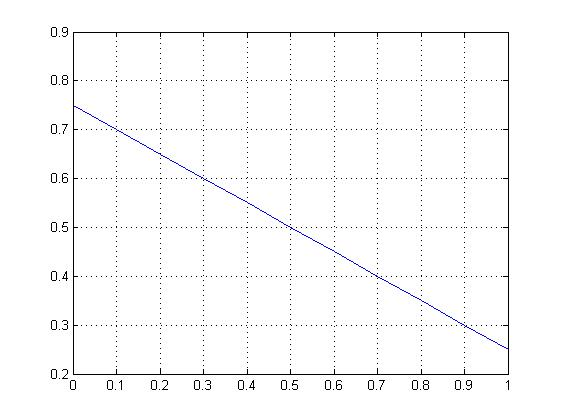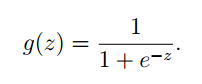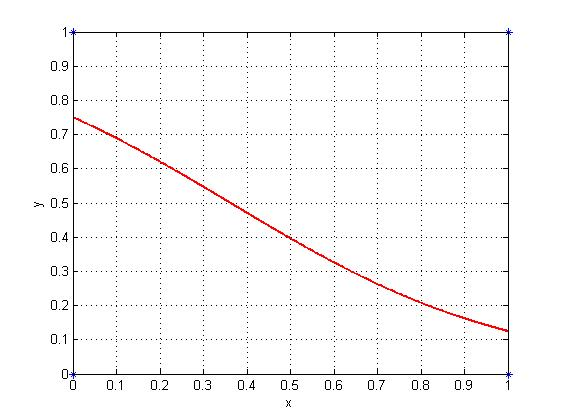# 机器学习引言

(在苹果系统下，如果文章中的图片不能正常显示，请升级Safari浏览器到最新版本，或者使用Chrome、Firefox浏览器打开。)

机器学习是人工智能的一个分支，涉及概率论、统计学、逼近论、凸分析、计算复杂性理论等多门学科，已广泛应用于数据挖掘、计算机视觉、自然语言处理、生物特征识别、搜索引擎、医学诊断、检测信用卡欺诈、证券市场分析、DNA序列测序、语音和手写识别、战略游戏和机器人等领域。

—-WikiPedia.org

 X 0 1 0 0 1 0 1 1 Y 1 0 0 1 0 1 0 1

y = -0.5 * x + 0.75Figure 1Logistic Regression的函数定义如下：

hθ(x) = g(θT x)Figure 2

【机器学习 周志华】清华大学出版社

【数学之美 吴军】人民邮电出版社

【机器学习 – 维基百科】

【余弦相似性 – 维基百科】

【聚类分析 – 维基百科】

【推荐系统 – 维基百科】

MATLAB代码

1.

```X=[0 1 0 0 1 0 1 1];
Y=[1 0 0 1 0 1 0 1];
plot(X,Y,'o');
p=polyfit(X,Y,1);
x=[0:.1:1];
y=p(1)*x+p(2);
plot(x,y)
grid on;
```

2.

```x=[0 1 0 0 1 0 1 1]';
y=[1 0 0 1 0 1 0 1]';
st_ = [0.5];
ft_ = fittype('1/(1+7*exp(-r*(x-1))) ' ,...
'dependent',{'y'},'independent',{'x'},...
'coefficients',{'r'});
[cf_ ,good]= fit(x,y,ft_ ,'Startpoint',st_)
h_ = plot(cf_,'fit',0.99); %置信系数0.99
legend off;  % turn off legend from plot method call
set(h_(1),'Color',[1 0 0],...
'LineStyle','-', 'LineWidth',2,...
'Marker','none', 'MarkerSize',6);
hold on,plot(x,y,'*')
grid on
```

 版权声明本博客的文章除特别说明外均为原创，本人版权所有。欢迎转载，转载请注明作者及来源链接，谢谢。本文地址: https://blog.ailemon.me/2017/02/02/introduction-to-machine-learning/ All articles are under Attribution-NonCommercial-ShareAlike 4.0## “机器学习引言”上的6条回复ailemon说：平忠源说：平忠源说：